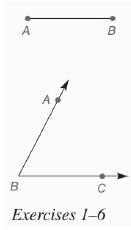Chapter 7.CR, Problem 1CRElementary Geometry For College St...

7th Edition
Alexander + 2 others
ISBN: 9781337614085

Solutions

Chapter
SectionElementary Geometry For College St...

7th Edition
Alexander + 2 others
ISBN: 9781337614085
Textbook Problem

In Review Exercises 1 to 6, use the figure shown.Construct a right triangle so that one leg has length A B and the other leg has length twice A B .To determine

To construct: A right triangle so that one leg has length AB and the other has length twice AB.

Explanation

Given:

The given figure is shown below:

Calculation:

Draw the horizontal line AB.

Draw other line which is twice of AB.

Now, set the compass width to the length of AC which is same as twice of AB then with that compass width move it to point A draw an arc above A and join AC...

Still sussing out bartleby?

Check out a sample textbook solution.

See a sample solution

The Solution to Your Study Problems

Bartleby provides explanations to thousands of textbook problems written by our experts, many with advanced degrees!

Get Started

limx0xex=1

Calculus: Early Transcendentals

Expand each expression in Exercises 122. x(4x+6)

Finite Mathematics and Applied Calculus (MindTap Course List)

In Exercises 33-38, rewrite the expression using positive exponents only. 37. 120(s + t)3

Applied Calculus for the Managerial, Life, and Social Sciences: A Brief Approach

Evaluate the integral. 03/2sinxdx

Single Variable Calculus: Early Transcendentals

Graph each of the following lines. y=12x

Trigonometry (MindTap Course List)

Using for |x| < 1 and differentiation, find a power series for .

Study Guide for Stewart's Single Variable Calculus: Early Transcendentals, 8th